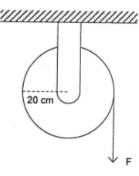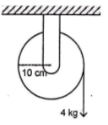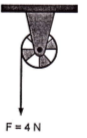# Dynamics of rotational motions – problems and solutions

1. A pulley with the moment of inertia I = 2/5 MR2 has a mass of 2-kg. If the moment of force on the pulley is 4 N.m then what is the linear acceleration of the pulley. Acceleration due to gravity is g = 10 m.s-2.

Known :The moment of inertia of the pulley (I) = 2/5 MR2

Mass of pulley (M) = 2 kg

Moment of force (τ) = 4 Nm

Acceleration due to gravity (g) = 10 m.s-2

Radius of pulley (R) = 20 cm = 0.2 m

Wanted : The linear acceleration (a)

Solution :

The moment of inertia of pulley (I) :

I = 2/5 MR2 = 2/5 (2)(0.2)2 = 2/5 (2)(0.04) = 2/5 (0.08) = 0.16 / 5 = 0.032 kg m2

(α) :

τ = I α

α = τ/I = 4 / 0.032 = 125 rad.s-2

The linear acceleration (a) :

a = R α = (0.2)(125) = 25 m.s-2

The linear acceleration of the edge of pulley is 25 m.s-2.

[irp]

2. Acceleration of pulley is 2 ms-2. Acceleration due to gravity is g = 10 m.s-2. What is the moment of inertia of the pulley.

Known :

The linear acceleration of the edge of pulley (a) = 2 ms-2Acceleration due to gravity (g) = 10 m.s-2

Radius of pulley (R) = 10 cm = 0.1 m

Object’s weight (w) = m g = (4)(10) = 40 N

Wanted : The moment of inertia of pulley

Solution :

The moment of force (τ) :

τ = F R = w R = (40)(0.1) = 4 Nm

The angular acceleration of the pulley (α) :

a = R α

α = a/R = 2 / 0.1 = 20 rad.s-2

The moment of inertia of the pulley (I) :

τ = I α

I = τ/α = 4/20 = 0.2 kg m2

3. The pulley accelerated at 1 ms-2. If radius of pulley is 10 cm,what is the moment of inertia of the pulley.

Known :

The linear acceleration of the edge of pulley (a) = 1 ms-2Acceleration due to gravity (g) = 10 m.s-2

Radius of pulley (R) = 10 cm = 0.1 m

Force (F) = 4 N

Wanted : The moment of inertia of the pulley

Solution :

The moment of force (τ) :

τ = F R = (4)(0.1) = 0.4 Nm

The angular acceleration of pulley (α) :

a = R α

α = a/R = 1 / 0.1 = 10 rad.s-2

The moment of inertia of pulley (I) :

τ = I α

I = τ/α = 0.4 / 10 = 0.04 kg m2

[irp]